# ConvBERT: Improving BERT with Span-based Dynamic Convolution

## ConvBERT

### Motivation

$$\operatorname{Self}-\operatorname{Attn}(Q, K, V)=\operatorname{softmax}\left(\frac{Q^{\top} K}{\sqrt{d_{k}}}\right) V$$

#### Parameters Redundancy

Self - Attention在处理问题时可能会存在参数冗余的问题: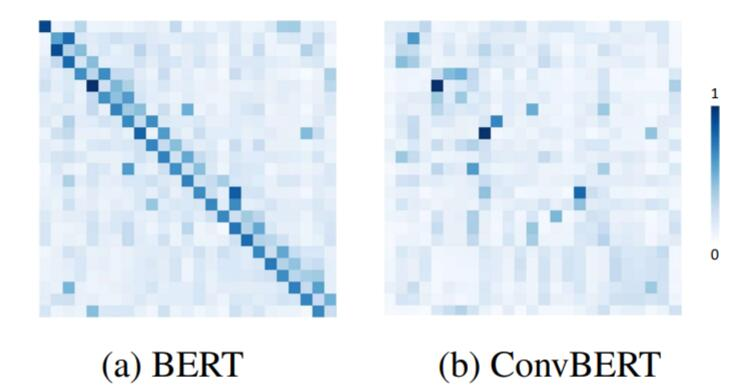#### Convolutional based Attention

$$\operatorname{LConv}(X, W, i)=\sum_{j=1}^{k} W_{j} \cdot X_{\left(i+j-\left\lceil\frac{k+1}{2}\right\rceil\right)}$$

$$\operatorname{DConv}\left(X, W_{f}, i\right)=\operatorname{LConv}\left(X, \operatorname{softmax}\left(W_{f} X_{i}\right), i\right)$$

### Span - based Dynamic Convolution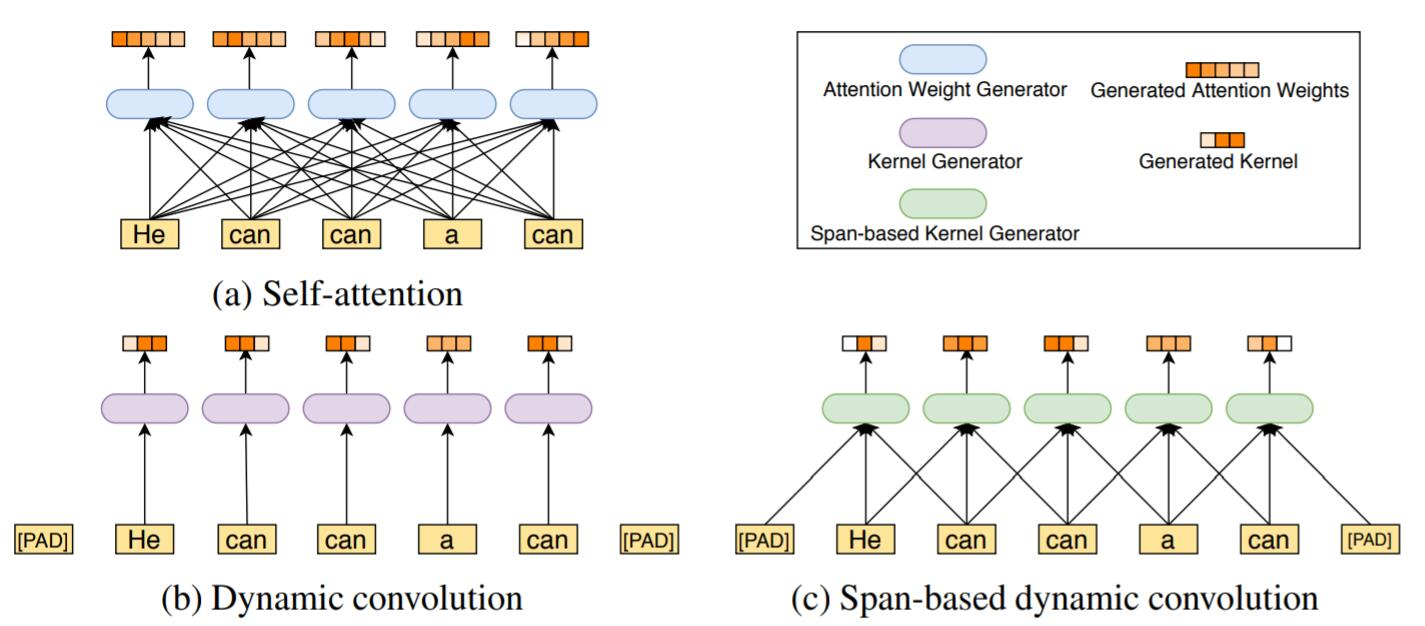$$f\left(Q, K_{s}\right)=\operatorname{softmax}\left(W_{f}\left(Q \odot K_{s}\right)\right)$$

$\odot$ 为逐元素点乘, $W_f$ 为可训练的权重矩阵.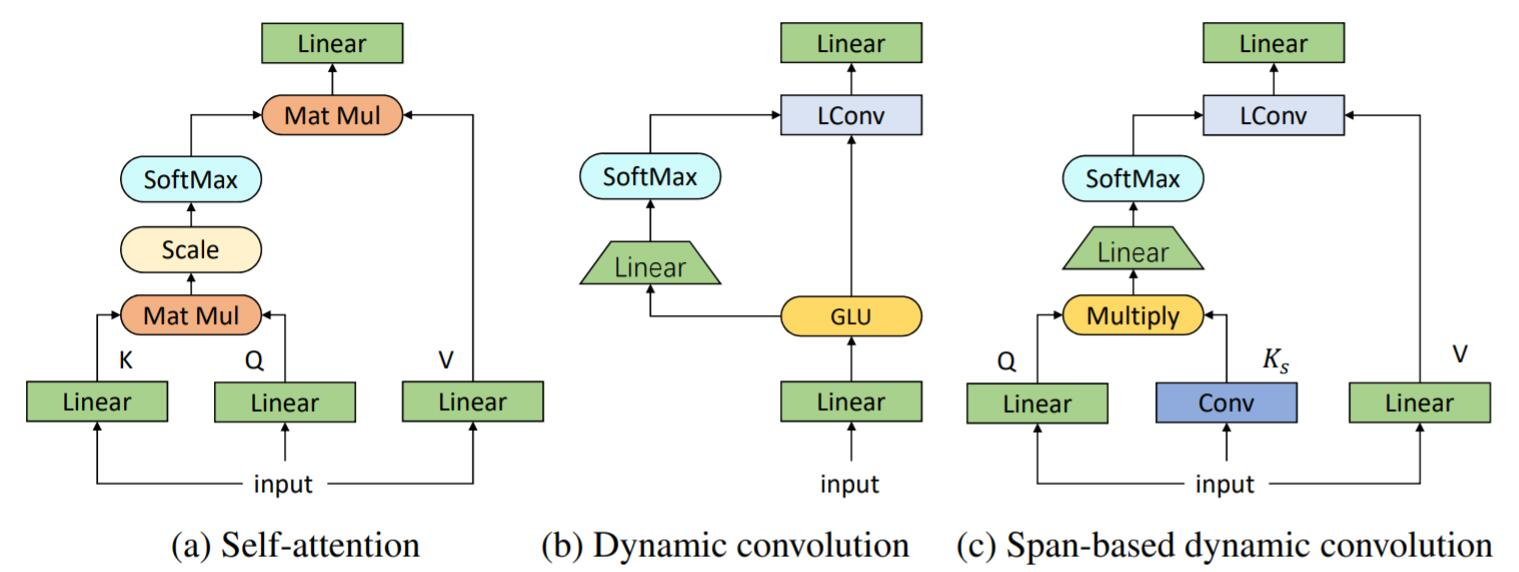$$\operatorname{SDConv}\left(Q, K_{s}, V ; W_{f}, i\right)=\operatorname{LConv}\left(V, \operatorname{softmax}\left(W_{f}\left(Q \odot K_{s}\right)\right), i\right)$$

### ConvBERT Architecture

#### Mixed Attention

$$\text {Mixed-Attn}\left(K, Q, K_{s}, V ; W_{f}\right)=\operatorname{Cat}\left(\text {Self-Attn}(Q, K, V), \operatorname{SDConv}\left(Q, K_{s}, V ; W_{f}\right)\right)$$

#### Bottleneck Design for Self - Attention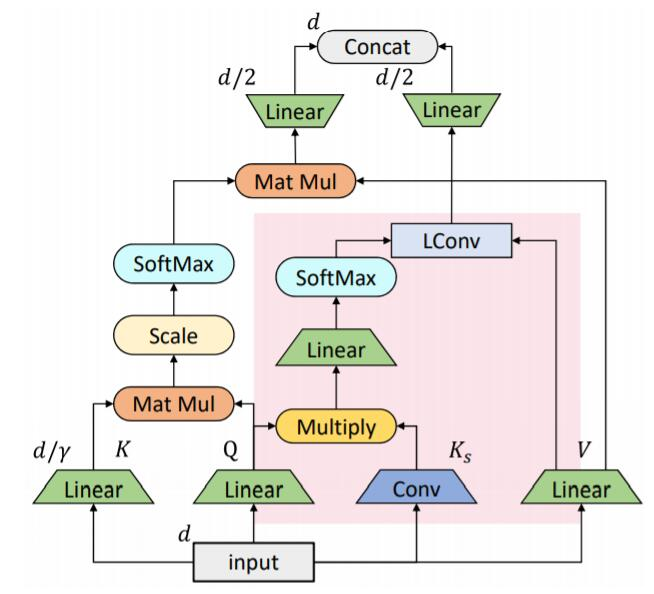#### Grouped Feed - Forward Module

$$\displaylines{ M=\Pi_{i=0}^{g}\left[f_{\frac{d}{g} \rightarrow \frac{m}{g}}^{i}\left(H_{\left[:, i-1: i \times \frac{d}{g}\right]}\right)\right], M^{\prime}=\operatorname{GeLU}(M) \\ H^{\prime}=\Pi_{i=0}^{g}\left[f_{\frac{m}{g} \rightarrow \frac{d}{g}}^{i}\left(M_{\left[:, i-1: i \times \frac{m}{g}\right]}^{\prime}\right)\right] }$$

## Experiments

### Ablation Study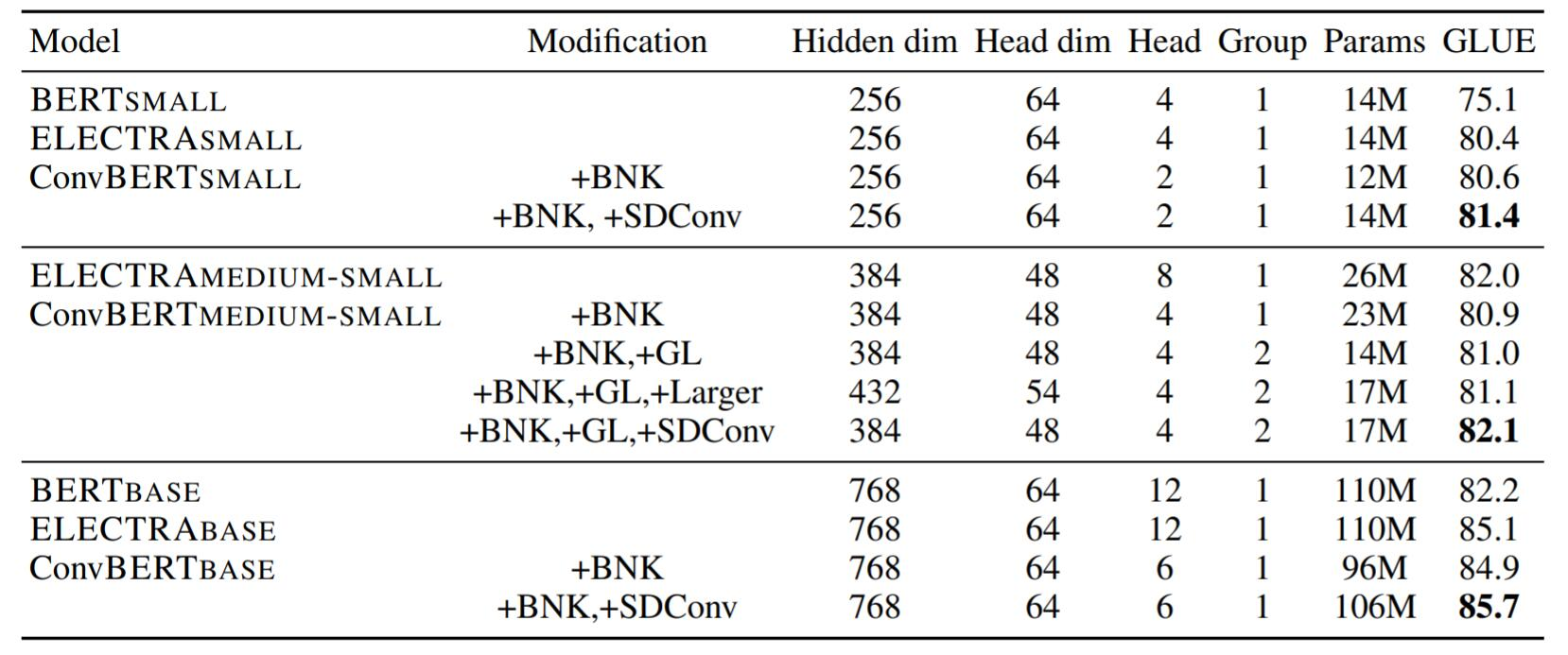#### Kernel Size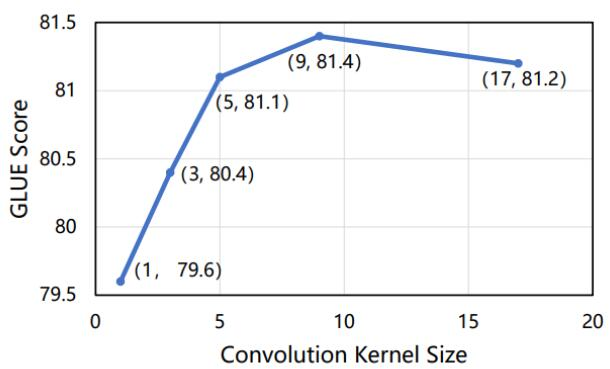#### Ways to integrate convolution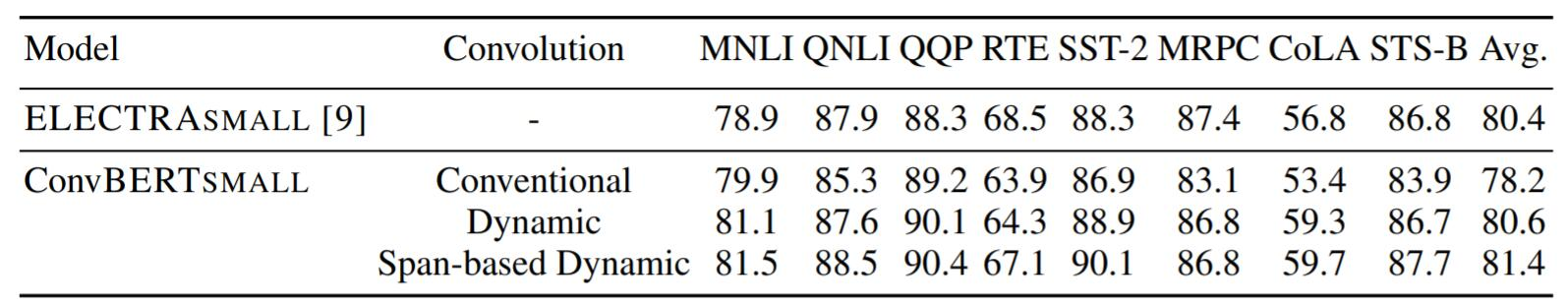### Comparison results

#### GLUE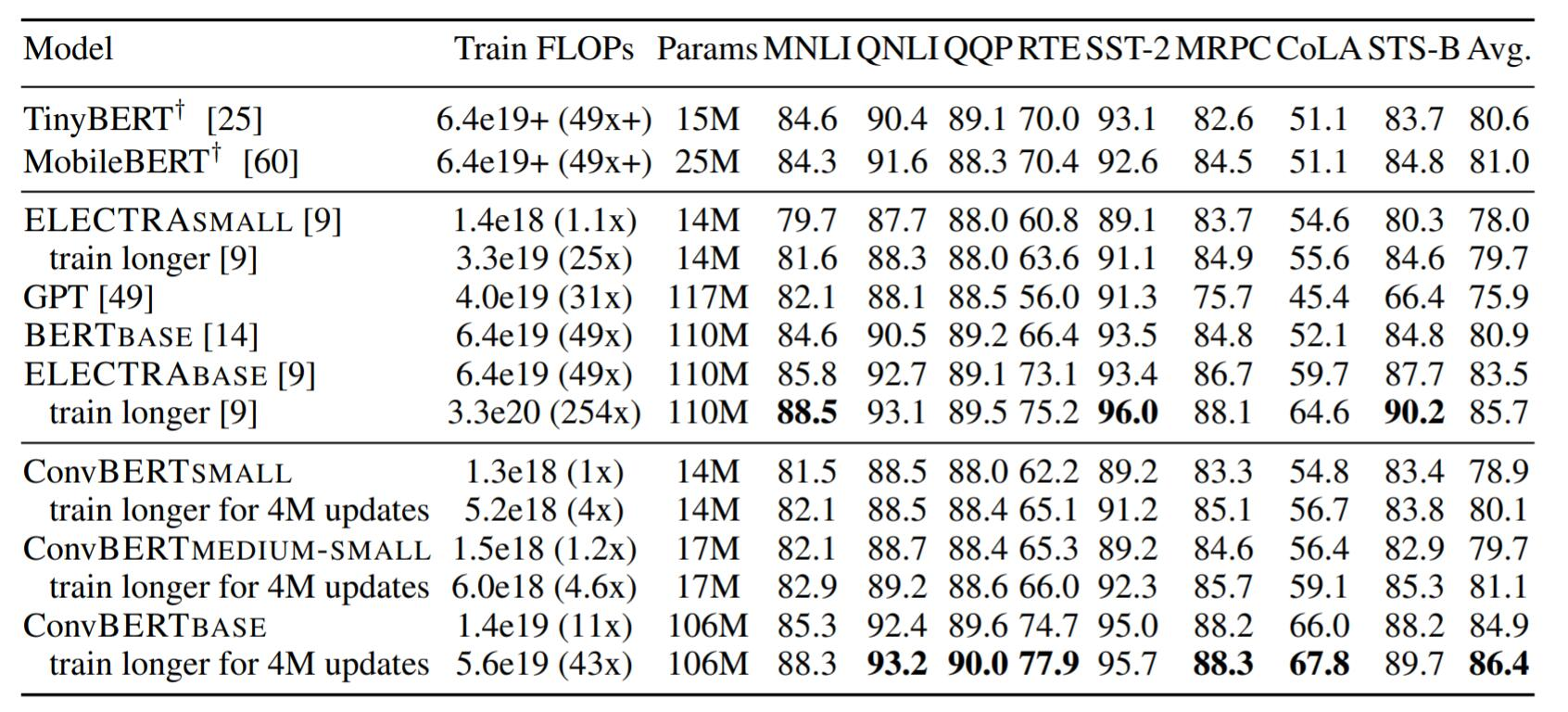ConvBERT在低资源的情况下结果良好.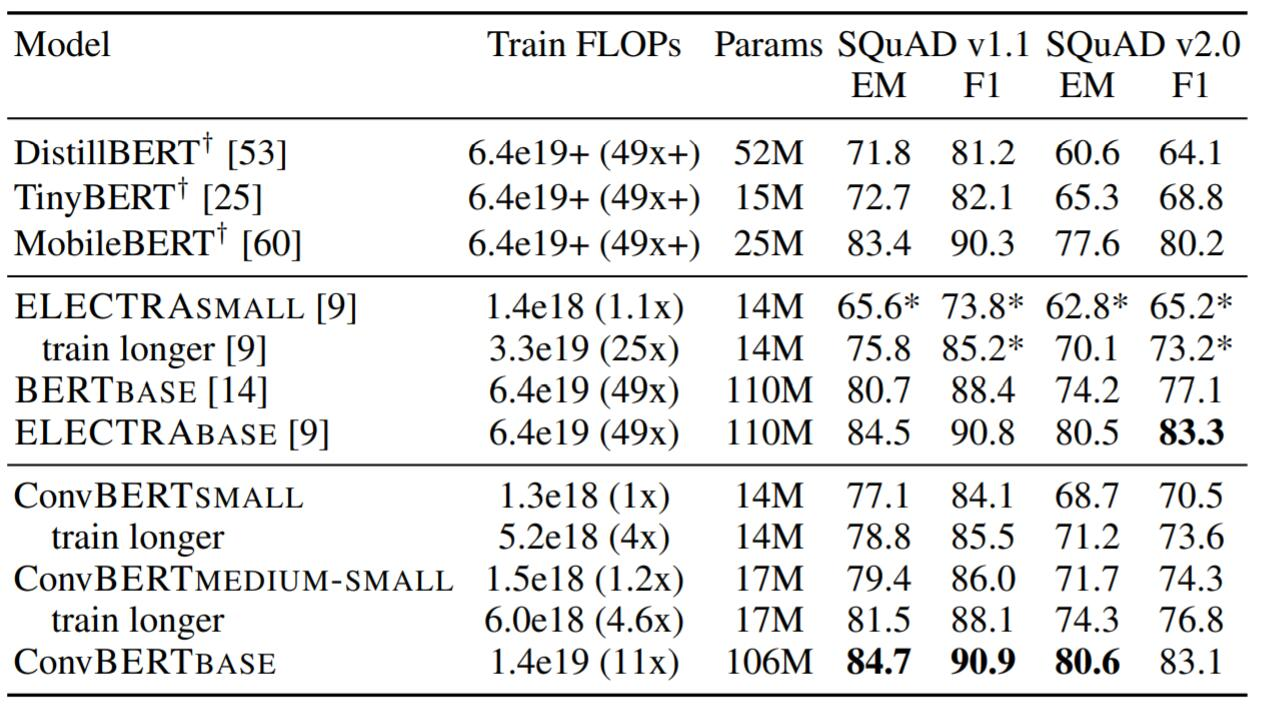## Summary

上一篇Introduction: Graph Neural Network

2021-03-04PPKE: Knowledge Representation Learning by Path-based Pre-training

2021-01-18
目录